Courses

# Test: Heat Transfer By Forced Convection - 2

## 10 Questions MCQ Test Heat Transfer | Test: Heat Transfer By Forced Convection - 2

Description
This mock test of Test: Heat Transfer By Forced Convection - 2 for Chemical Engineering helps you for every Chemical Engineering entrance exam. This contains 10 Multiple Choice Questions for Chemical Engineering Test: Heat Transfer By Forced Convection - 2 (mcq) to study with solutions a complete question bank. The solved questions answers in this Test: Heat Transfer By Forced Convection - 2 quiz give you a good mix of easy questions and tough questions. Chemical Engineering students definitely take this Test: Heat Transfer By Forced Convection - 2 exercise for a better result in the exam. You can find other Test: Heat Transfer By Forced Convection - 2 extra questions, long questions & short questions for Chemical Engineering on EduRev as well by searching above.
QUESTION: 1

Solution:
QUESTION: 2

### Nusselt number in case of forced convection is the function of

Solution:

For forced convection
Nu - f(Re, Pr)

QUESTION: 3

### For laminar flow in a tube for constant heat flux condition Nusselt number is approximately equal to

Solution:
QUESTION: 4

For laminar flow in a tube for constant wall temperature Nusselt number is approximately equal to

Solution:
QUESTION: 5

If Nusselt number is 400, Stanton number is 0.5 and Reynold’s number is 40, then Prandtl number will be

Solution: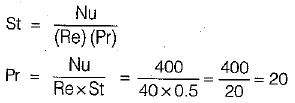QUESTION: 6

The velocity and temperature distribution in a pipe flow are given by U(r) and T(r). If um is the mean velocity at any section of the pipe, the mean temperature at that section is

Solution: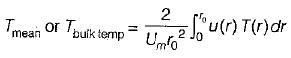QUESTION: 7

A fluid flowing over aflat plate has the following properties:
Dynamic viscosity = 25 x 10-6 kg/ms
Specific heat = 2 kJ/kgK
Thermal conductivity = 0.05 W/mK

The hydrodynamic boundary layer thickness found to be 0.5 mm. The thickness of thermal boundary layer would be

Solution: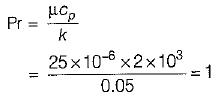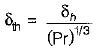Hence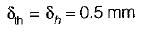QUESTION: 8

For a laminar flow of air (Pr = 0.71) over a flat plate at zero incidence, the thickness of the velocity boundary layer δ and the hydrodynamic boundary layer δt are such that

Solution: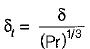Since,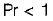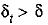QUESTION: 9

Heat is lost from a 100 mm diameter steam pipe placed horizontally in ambient at 30°C. If the Nusseit number is 25 and thermal conductivity of air is 0.03 W/mK then the heat transfer coefficient will be

Solution: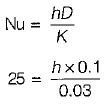or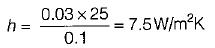QUESTION: 10

Heat transfer coefficients for free convection in gases, forced convection in gases and vapours and for boiling water lie respectively in the range of

Solution: